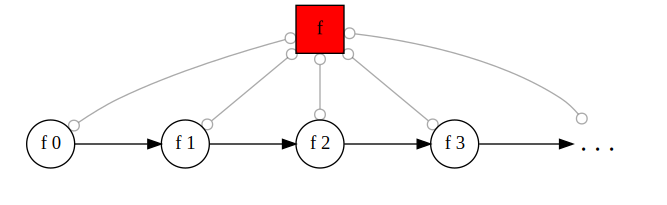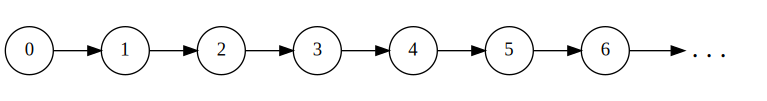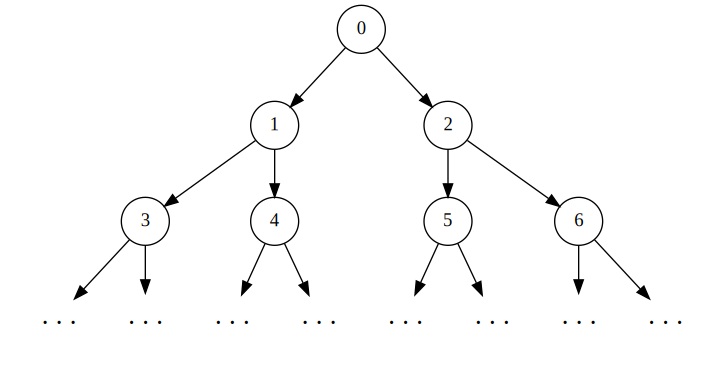# Memoization via Representables

18 September 2022

What is the most basic container type a language can have? Some people may answer vectors, others would go with hash tables, but in this post I am arguing in favor of functions. Yes, functions. Even though they aren’t generally seem as a data structure per se, we will see that most containers are in fact a way to represent a function with a given storage layout. To illustrate this “functions are containers” idea, let’s take a look at an application that tightly couples both concepts: memoization.

By the way, in case this is the first time you hear about it: memoization is a programming technique where instead of letting a function calculate the same value whenever it is called, we instead store the already calculated value somewhere and just do a lookup instead of redoing the entire calculation.

What I like the most in this post’s code is that we’re going to delve deeply into the realm of abstraction to then emerge with a concept with clear and practical applications!

{-# LANGUAGE DeriveFunctor,       TypeFamilies        #-}
{-# LANGUAGE ScopedTypeVariables, RankNTypes          #-}
{-# LANGUAGE TypeApplications,    AllowAmbiguousTypes #-}
import Numeric.Natural
import Data.Kind (Type)

## Sequences are functions that do not forgetAn important fact that is normally briefly alluded in any mathematics book and immediately forgotten by (almost) every reader is that whenever you see a subindex such as x_n, what it in fact denotes is a function application x(n).1 Now consider the datatype of infinite streams as in the previous post:

data Stream a = a :> Stream a
deriving Functor

infixr 5 :>

This type models sequences of type a, the kind of thing a mathematician would denote as \{x_n\}_{n \in \mathbb{N}}. Oh look, there’s a subindex there! Our previous discussion tells us that we should be able to interpret a Stream a as a function Natural -> a. This is done by indexing: we turn a stream xs into the function that takes n : Natural to the nth element of xs. The definition is recursive, as one might expect:

-- Access the nth value stored in a Stream
streamIndex :: Stream a -> (Natural -> a)
streamIndex (x :> _)  0 = x
streamIndex (_ :> xs) n = streamIndex xs (n-1)

Conversely, given any f : Natural -> a, we can form a stream by applying f to each natural number to form something like [f 0, f 1, f 2, f 3,..., f n,...]. Since Stream is a functor, we achieve this by mapping f into the stream of natural numbers:

-- Take f to [f 0, f 1, f 2, f 3, ...]
streamTabulate :: (Natural -> a) -> Stream a
streamTabulate f = fmap f naturals where
naturals = 0 :> fmap (+1) naturals

These functions are inverse to one another:

streamIndex    . streamTabulate = id
streamTabulate . streamIndex    = id

Meaning that we thus have a natural isomorphism Stream a ≃ Natural -> a. This is a strong assertion and means that, mathematically speaking, Streams and functions from the Naturals are essentially the same thing. We are doing Haskell in here, however, not pure math. And in a programming language meant to run in a real computer, not only in the realm of ideas, we also must take into account something more: how are those types laid out into memory?

In the case of functions, they are compiled to chunks of instructions that calculate some value. Specially, if you have some rather expensive function f : Natural -> a and have to calculate f n in many parts of your program for the same n, all work will have to be redone each time to get that sweet value of type a.2 Streams, on the other hand, are lazy infinite lists and, because of Haskell’s call-by-need evaluation strategy, its components remain saved into memory for reuse.3

This last paragraph is the heart of memoization in Haskell: one does not memoize functions, one turns functions into data and the language automatically memoizes the data. I don’t know about you, but I find this very cool indeed.

## Memoizing with recursion

A large inspiration to this post comes from the great introduction to memoization in the Haskell wiki. Thus, we will follow their lead and explore the Fibonacci sequence as a recurring example throughout this post.

I must admit that I find illustrating a recursion concept through the Fibonacci numbers kind of a cliché… Nevertheless, clichés have their upside in that you, the reader, will have seen them so much that may even perhaps feel familiar with what we will be doing here. The Fibonacci numbers are also a well-known example where memoization can make a function go from exponential to polynomial complexity. Well, let’s start with their usual recursive definition:

fibRec :: Num a => Natural -> a
fibRec 0 = 0
fibRec 1 = 1
fibRec n = fibRec (n-1) + fibRec (n-2)

Although elegant, this definition is extremely slow. Running fibRec 100 on ghci already took much longer than I was disposed to wait… The problem is that the recursion has to calculate the same arguments a lot of times, leading to an exponential complexity.

Since the problem is overlapping calculations, we can accelerate this function using memoization. But in this case, just turning it into a stream is not enough, because the fibRec will still use the slow definition to build each of the sequence’s component. But fear nothing, there is a salvation! It starts by writing the function in operator form, instead of using recursion, just like we did with the Bellman Equation in my previous post about dynamic programming.

fibOp :: Num a => (Natural -> a) -> (Natural -> a)
fibOp v 0 = 0
fibOp v 1 = 1
fibOp v n = v (n-1) + v (n-2)

You can think of fibOp as one step of the Fibonacci recursion, where v is a function that knows how to continue the process. Another way to look at it, that is closer to the dynamic programming view, is that if v is an estimation of the Fibonacci values then fibOp v will be an improved estimation given the available information. No matter what view you choose, the important part to us is that the fixed point of fibOP is fibRec.

fix f = let x = f x in x

fibNaive :: Num a => Natural -> a
fibNaive = fix fibOp  -- same as fibRec

Where we called it fibNaive because it would be rather naive to do all this refactoring in order to arrive at the exact same thing…

Alright, with fix we have all the necessary building blocks to accelerate our calculations. It’s now time to fit them together! Before fixing the operator, we will turn it into something that “keeps a memory”. If we compose our tabulation function with fibOp, we get a function turns a function v into a Stream, over which we can index to get back a function. In this case, however, the same stream is shared for all arguments. Thus, the fixed point indexes into this Stream during the recursion process! Moreover, there is nothing specific to the Fibonacci sequence in this process, so we can abstract this procedure into a separate function.

streamMemoize :: ((Natural -> a) -> Natural -> a) -> Natural -> a
streamMemoize f = fix (streamIndex . streamTabulate . f)

fibSmart :: Num a => Natural -> a
fibSmart = streamMemoize fibOp

Notice that by our previous discussion, streamIndex . streamTabulate equals id. Thus, by construction, fibNaive and fibMemo are also equal as functions. Nevertheless, their runtime behavior is considerably different! As Orwell would put it: in terms of execution, some equals are more equal than others.

## A Call for Representation

Very well, What is a function k -> a after all? The textbook definition says it is a rule that for each element of type k associates a unique element of type a. The previous examples have shown us that there are data structures which, in the sense above, behave a lot like functions. For example, we saw how to convert between Streams and functions and even used it to accelerate the calculation of recursive functions. In the case of Streams, both streamIndex and streamTabulate are natural transformations4, meaning that there is a natural isomorphism between streams and functions with Natural as domain:

forall a. Stream a ≃ Natural -> a.

We call a functor isomorphic to a type of functions, a Representable Functor. Those have important applications in Category Theory because they are closely related to universal properties and elements. However, today we are interested in their more mundane applications, such as memoizing domains other than the Naturals.

In Haskell, we can codify being Representable as a typeclass. It must have an associated type saying to which function type the Functor is isomorphic, together with two natural transformations that witness the isomorphism.

class Functor f => Representable f where
type Key f :: Type
tabulate   :: (Key f -> a) -> f a
index      :: f a          -> (Key f -> a)
-- Class laws:
-- index    . tabulate = id
-- tabulate . index    = id

As you can imagine, there is a Representable instance for Streams using what we have defined in the previous sections.

instance Representable Stream where
type Key Stream = Natural
index    = streamIndex
tabulate = streamTabulate

Another interesting instance is for functions themselves! After all, the identity is, strictly speaking, a natural isomorphism.

instance Representable ((->) k) where
type Key ((->) k) = k
index    = id
tabulate = id

With some type-level magic, we can write a generalized memoization procedure. It has a scarier type signure, since we’re striving for genericity, but the idea remains the same: precompose with tabulate and index before fixing. The function is essentially the same we wrote before for Streams for parameterized on our Representable Functor of choice.

-- | Memoize a recursive procedure using a Representable container of your choice.
memoize :: forall f a. Representable f => ((Key f -> a) -> (Key f -> a)) -> (Key f -> a)
memoize g = fix (index @f . tabulate . g)

We can recover our Stream-memoized Fibonacci by telling memoize that we choose Stream as the container:

fibSmart' :: Num a => Natural -> a
fibSmart' = memoize @Stream fibOp

The function above is the same as our “more manual” fibSmart from before. As a matter of fact, even the naive recursion is case of these memoization schemes! By using the Representable instance of functions, the methods do nothing, and we get a memoization scheme that has no storage. Well, this is equivalent to our naive approach from before.

fibNaive' :: Num a => Natural -> a
fibNaive' = memoize @((->) Natural) fibOp

## Speeding up the Fibonacci even more

With our Representable machinery all set, it would be a shame to end this post with just one example of memoization.5 So, let’s see how we can memoize the Fibonacci function using an infinite binary tree structure. This is a fun example to look at because the isomorphism is not as straightforward as with Streams and because it is much faster. We begin by defining our datatype.

data Tree a = Node a (Tree a) (Tree a)
deriving Functor

By now, you should already know how the memoization works at a high-level, so we can just define it as before.

fibTree :: Num a => Natural -> a
fibTree = memoize @Tree fibOp

Alright, how are these Trees representable? In the case of Streams, the relation was clear: we kept decreasing the index and advancing on the Stream until the index was zero. And this amounted to recursing on a unary representation of the Naturals: we advanced at a successor and stopped at zero. The secret to translate this idea to Trees is to look at a natural number as written in binary. I personally find this relation easier to explain with a drawing. So, while we index Streams with a linear approach,For Trees, we index using a breadth-first approach.By the way, I don’t know if the figure above makes it clear since we are starting with zero, but this arrangement is equivalent to branching on the based on the number’s evenness. We descend left on odd numbers and right on even numbers. The crucial part of this representation is that we are able to reach the n-th index in O(log n) steps, instead of the n steps required for the Stream. Alright, it’s time to turn all this talking into a proper instance!

Let’s begin with some helpers to make the evenness check more explicit.

data Eveness = Even | Odd

evenness :: Integral a => a -> Eveness
evenness n = if odd n then Odd else Even

instance Representable Tree where
type Key Tree = Natural

We tabulate a function using the same ideas as for Streams: create a Tree of natural numbers and map the function over it. The tree is created by branching into even and odd numbers.

 tabulate f = fmap f nats where
nats = Node 0
(fmap (\ n -> 2*n + 1) nats)
(fmap (\ n -> 2*n + 2) nats)

For indexing, we test for the number’s evenness and branch accordingly until we’re looking for the zeroth index.

 index (Node a _ _) 0 = a
index (Node _ l r) n = case evenness n of
Odd  -> index l (div n 2)
Even -> index r (div n 2 - 1)

## Farewell

With this we finish our stroll through memoization-land. By the way, this post is a literate haskell file. Thus, I really recommend you to take the functions in it and try some benchmarks to see how much the memoization helps. From my own tests in ghci, the Tree Fibonacci is much faster than the other two. But compiling with optimizations, the Stream approach gets much faster, so I might need a better benchmark in there.

1. I must comment, perhaps to the abhorrence of my Haskell readers, that I’m rather fond Fortran. One thing I like about it is how the language uses the same syntax to denote vector indexing and function application: x(n).↩︎

2. In fact, not all work must be necessarily redone. If we are calculating f n twice in the same context, the compiler may be smart enough to do some sharing and only calculate it once. Of course, this works in Haskell thanks to the magic of referential transparency.↩︎

3. Well, at least until the next gc pass if it is not in the top-level.↩︎

4. In Haskell, any polymorphic function h :: forall a. F a -> G a, where F, G are functors, is a natural transformation.↩︎

5. Well, two if you count the naive approach.↩︎